•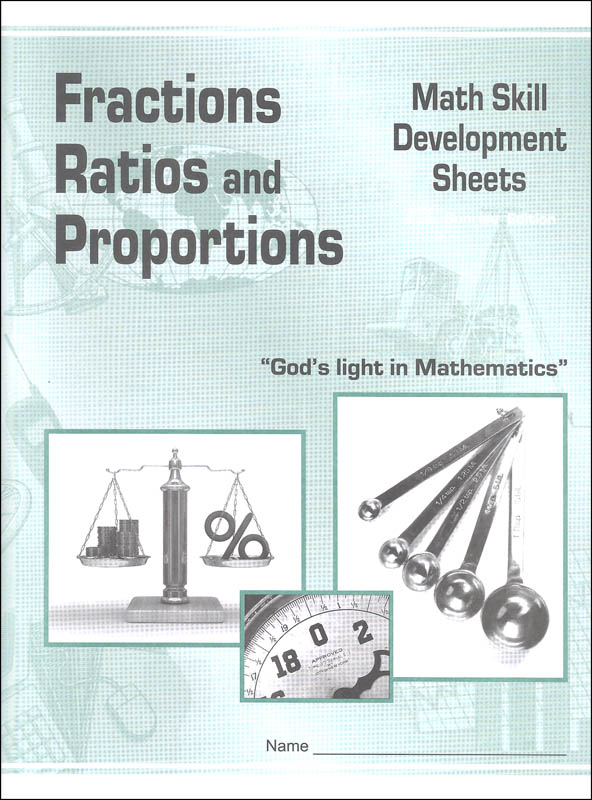•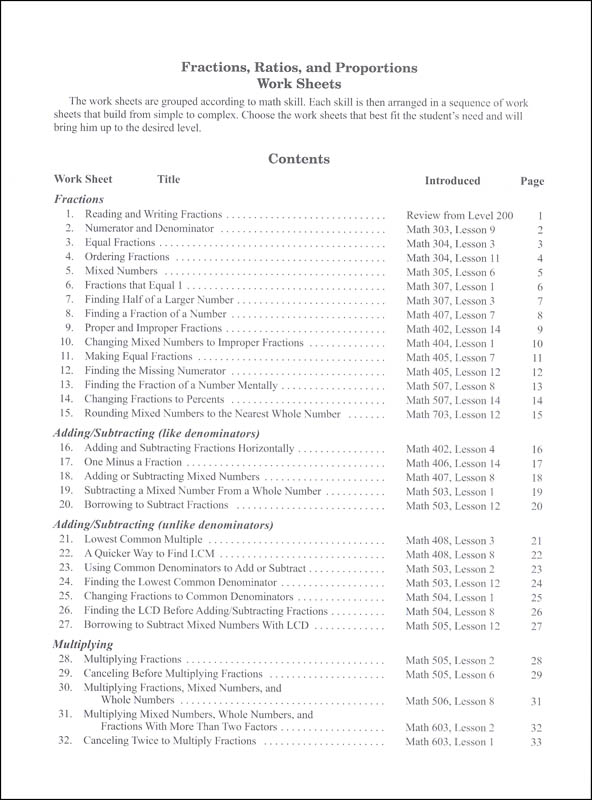•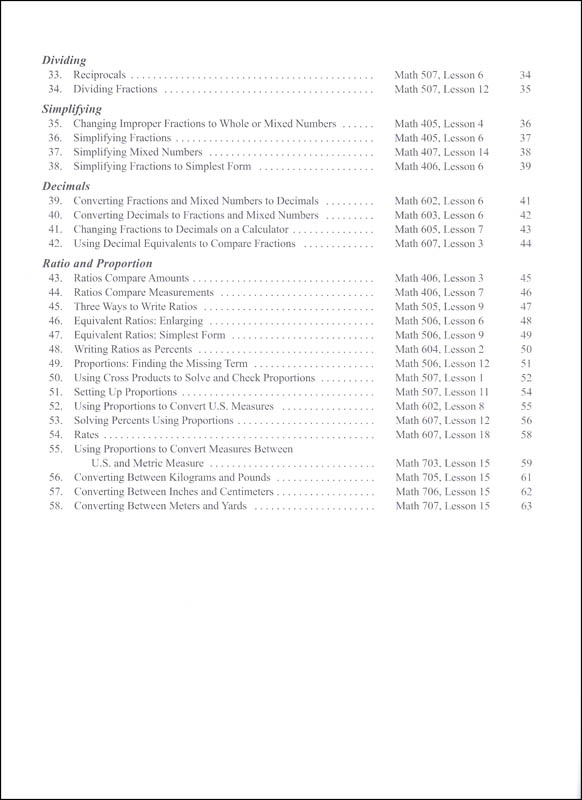•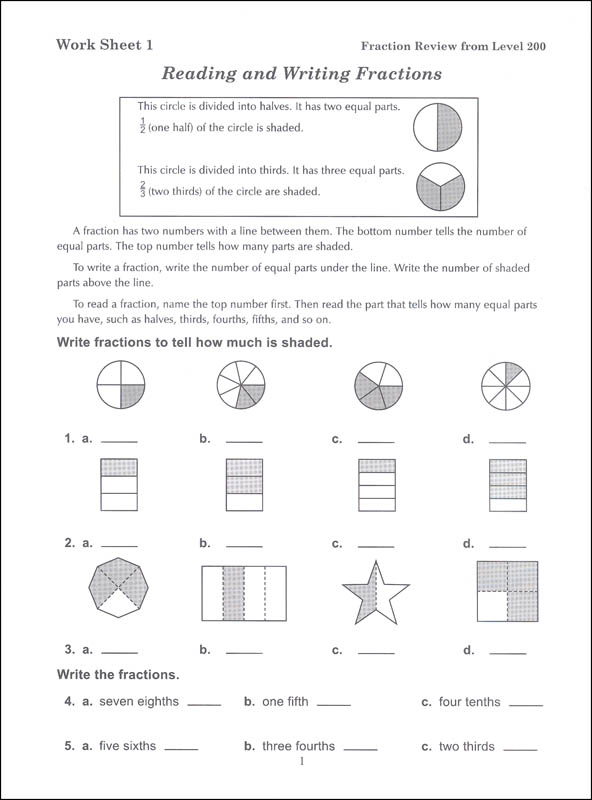•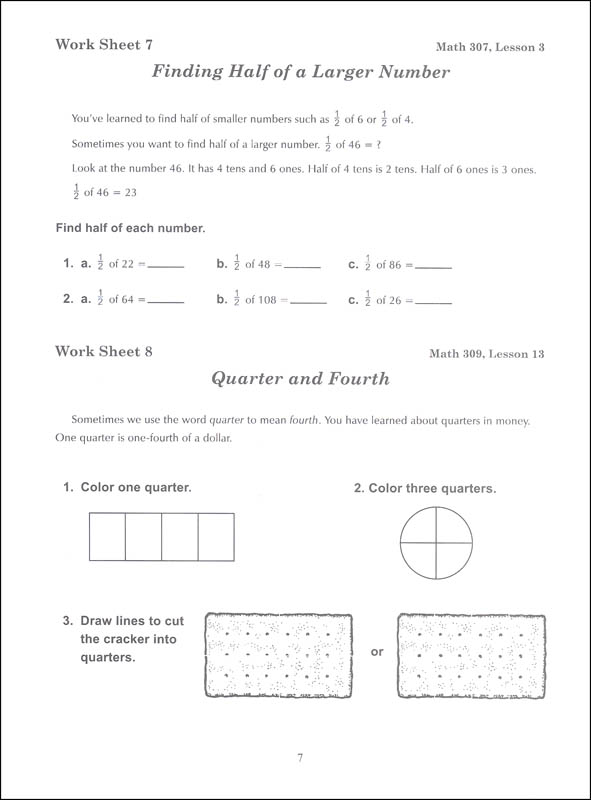# Fractions, Ratios, and Proportions Math Skill Development Worksheets

# 033634

Our Price: \$4.95
2 In Stock.
Qty:
Qty:

Item #: 033634 2-7

#### Product Description:

Designed to prepare students transitioning into the CLE Math curriculum, these skill-based worksheets provide any remedial practice or review for any math student who needs a little more time to master concepts. Beginning with a quick overview of the concept, each worksheet offers standalone practice related to the concept. Topics include an overview of fractions, adding and subtracting fractions with like and unlike denominators, multiplying and dividing fractions, simplifying fractions, decimals, ratios, proportions, and U.S and metric conversions. Topics are organized by skill and complexity. For students needing more instruction, the table of contents identifies the CLE math level and lesson where the concept is introduced. Worksheets are reproducible for the purchasing family or school. An answer key includes student worksheets with answers filled in. Package includes both the student worksheets and answer key. Staple bound. 64 pgs. ~Deanne

#### Publisher Description:

These worksheets cover concepts in fractions, ratios, and proportions as taught in CLE Sunrise Math, Levels 200 through 700. Worksheets familiarize students with CLE's math terminology and methods and help fill in any gaps for students coming in from another math curriculum. Teachers choose sections of the workbook that the student needs to bring him up to mastery level for his grade. Reproducible for classroom use, but not for sale or mass distribution.

Category Description for Christian Light Mathematics:

To help ensure students learn their math facts, daily speed drills, oral exercises, and flash-cards are tools used for students in grades 1-4. Basic algebraic and geometric concepts are introduced early, along with money, problem solving, mental math, and number patterns to help students become proficient in these skills. A few supplemental materials are needed for the lower grades Grade 1 uses addition and subtraction flash cards (#72144); My Counting Book (#72357); Counting Chart (#72173); and optional My Calendar Book (#21786); and student clock (#15339 or #60101); Grade 2 uses addition and subtraction flash cards (#72144); Counting Chart (#72173); and optional multiplication/division flash cards (#72356); My Calendar Book (#21786); student clock (#15339 or #60101); and math reference chart (#68518); Grade 3 uses optional flash cards as in 1st and 2nd grades, and the math reference chart. Grades 4, 5, and soon to be 6 (later in 2018) offer the option of using the Light Unit approach or text book approach same content but a choice of consumable or non-consumable.

If you choose the Light Units path, parents need only to purchase the Light Units and Teacher Guides or answer keys. If choosing the textbook path, then you will need the textbook (non-consumable), student pack (consumable), Teacher Guide and/or Full Solutions Answer Key. Textbooks are colorful with illustrations and pictures, and provide opportunity for students to do their work on a separate sheet of paper. The Student Pack includes quizzes, tests, and daily speed/mastery drills (math facts review) and measurement equivalents. Full Solutions Answer Key shows each problem of the textbook, quizzes, and tests with step-by-step solutions. Teacher Guides for the textbook are also in full color and include student pages with answers and a cd of printables posters, charts, and practice sheets.

The addition/subtraction flash cards accompanying this curriculum for grades 1-4 are numbered and tabbed to correspond to the teacher guides at those grade levels. Cards measure 3.5" x 7.5", addition/subtraction go from 0 to 18. Multiplication/division flash cards measure 3.5" x 5" and cover math facts from 0-12. The multiplication fact is on the front of the card with the opposite division fact on the reverse. These aren't numbered and tabbed like the addition/subtraction cards. Math Reference Charts are available for grades 2-6, 5-8, and 7/8. These are 8.5" x 11" laminated sheets for easy reference at a glance for those items that students need to remember but need a little help measurement tables, formulas, etc.

Grade 8 is considered pre-algebra and prepares students for any algebra 1 course. It introduces students to concepts needed at the higher levels of math such as Pythagorean theorem, irrational square roots, and basic trigonometry.

The sequence for high school is Algebra 1, Geometry, Algebra II, and Functions and Trigonometry. To use Algebra I you will need the 10 Light Units (students write on their own paper), solution key (solutions to the Light Units exercises), tests & quizzes, and the test & quiz answer key. These are all sold separately for the Algebra I level. Geometry, Algebra II, and Functions and Trigonometry, each use 10 Light Units and 5 answer keys. Finishing through Functions and Trigonometry will prepare students for college level math.

Consumer Math is an optional math course offered in this series. Equivalent to one high school credit, this course applies math to everyday situations. There are 10 Light Units and 5 answer keys needed to complete the course and they cover basic and everyday math fractions, decimals, percent, family finances, construction & building trades, service occupations, transportation, business services, and occupational diagrams. ~ Donna

Category Description for COMPREHENSIVE PROGRAMS - ALL GRADES:

Primary Subject
Mathematics
2
7
Format
Paperback
Brand Name
Christian Light
Weight
0.35 (lbs.)
Dimensions
11.0" x 8.12" x 0.19"